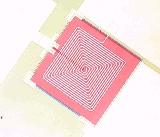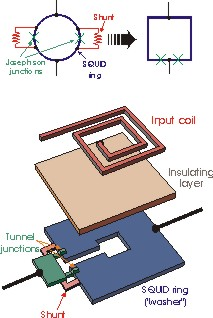Further Information
Home

Publications

 ez SQUIDSuperconducting Quantum Interference Devices (SQUIDs) Superconducting quantum interference devices (SQUIDs) are the most sensitive sensors for magnetic flux and magnetic field so far.  They consist of a small superconducting ring (less than 1 mm in diameter), which is interrupted by one or two so-called Josephson junctions, which essentially act as a "weak" superconductor.  Only a small current (on the order of microamps) can flow through such a weak link without dissipation.  Above a certain current, the so-called critical current Ic, a voltage drop develops across the junction.  A magnetic flux through the SQUID, induced by, e.g., an external magnetic field, alters the value of the critical current.  Specifically, it changes the phase of the Cooper pair wave function across the junction(s).  In the case of the so called direct current SQUID (dc SQUID, a superconducting ring with two junctions), the variation in critical current with varying magnetic flux can be detected by simply passing a dc current (hence the name dc SQUID) through the SQUID.  The value of this dc current is chosen to be slightly larger than the sum of the critical currents of the two Josephson junctions (the critical current of the SQUID).  Then a dc voltage drop develops across the SQUID, which varies periodically with varying magnetic flux.  The periodicity of this so-called flux-to-voltage transfer function of the SQUID is the flux quantum (approx. 2 fVs [femto volt-seconds]).  If the SQUID ring contains only a single junction (the so-called rf SQUID), it is usually coupled to a tuned circuit, consisting of an inductor in parallel with a capacitor, which is driven by an rf current.  The amplitude of the resulting rf voltage across this circuit oscillates with varying flux through the SQUID, similar to the oscillating dc voltage in case of the dc SQUID.  A citerion for the sensitivity of a SQUID is its energy resolution.  This is defined as the smallest change in energy which the SQUID can detect in a bandwidth of 1 Hz.  Typical values are 10-32 J/Hz for dc SQUIDs operated at 4 K.  This is not far away from the limit which is set by Heisenberg's uncertainty principle.  Although a SQUID primarily measures magnetic flux, it can measure all physical quantities which can be transformed into magnetic flux, such as electrical currents, voltages or displacements.  SQUIDs therefore can play an essential role in a large variety of applications, both in fundamental science and in industrial measurements.  Our SQUIDs are prepared in thin film technology (see figure below).  The SQUID ring is a square loop made of niobium.  The Josephson junctions are fabricated as tunnel junctions in a vertical geometry: A top niobium layer is separated from a bottom niobium layer (the SQUID ring) by a very thin aluminum oxide layer.  On top of the SQUID loop, we pattern a spiral coil which can be used to convert small electrical currents to magnetic flux, enabling the SQUID to also be used as a current detector or low or high-frequency amplifier.Optical-microscope image of a thin-film niobium dc SQUID.

 Blow-up view of a thin-film dc SQUID.ez SQUID Mess- und Analysegeräte Dr. Hans-Michael Mück, Herborner Strasse 9, 35764 Sinn, Germany email: info@ez-squid.de